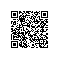# R语言中不能进行深度学习？

1.在后端安装带有张量的Keras。

2.使用Keras可以在R中构建不同类型的模型。

3.在R中使用MLP对MNIST手写数字进行分类。

4.将MNIST结果与Python中的等效代码进行比较。

5.结束笔记。

## 1.在后端安装带有TensorFlow的Keras。

install.packages("devtools")
devtools::install_github("rstudio/keras")

library(keras)

install_tensorflow()

install_tensorflow(gpu=TRUE)

2.使用keras可以在R中构建的不同类型的模型

1.多层感知器

2.卷积神经网络

3.循环神经网络

4.Skip-Gram模型

5.使用预先训练的模型，如VGG16，RESNET等

6.微调预先训练的模型。

## 3.使用R中的MLP对MNIST手写数字进行分类

#loading keras library
library(keras)
data<-dataset_mnist()
#separating train and test file
train_x<-data$train$x
train_y<-data$train$y
test_x<-data$test$x
test_y<-data$test$y
rm(data)
# converting a 2D array into a 1D array for feeding into the MLP and normalising the matrix
train_x <- array(train_x, dim = c(dim(train_x), prod(dim(train_x)[-1]))) / 255
test_x <- array(test_x, dim = c(dim(test_x), prod(dim(test_x)[-1]))) / 255
#converting the target variable to once hot encoded vectors using keras inbuilt function
train_y<-to_categorical(train_y,10)
test_y<-to_categorical(test_y,10)
#defining a keras sequential model
model <- keras_model_sequential()
#defining the model with 1 input layer[784 neurons], 1 hidden layer[784 neurons] with dropout rate 0.4 and 1 output layer[10 neurons]
#i.e number of digits from 0 to 9
model %>%
layer_dense(units = 784, input_shape = 784) %>%
layer_dropout(rate=0.4)%>%
layer_activation(activation = 'relu') %>%
layer_dense(units = 10) %>%
layer_activation(activation = 'softmax')
#compiling the defined model with metric = accuracy and optimiser as adam.
model %>% compile(
loss = 'categorical_crossentropy',
metrics = c('accuracy')
)
#fitting the model on the training dataset
model %>% fit(train_x, train_y, epochs = 100, batch_size = 128)
#Evaluating model on the cross validation dataset
loss_and_metrics <- model %>% evaluate(test_x, test_y, batch_size = 128)

4.MLP使用keras–R VS Python

#importing the required libraries for the MLP model
import  keras
from  keras.models  import Sequential
import  numpy  as  np
from  keras.datasets  import  mnist
(x_train, y_train), (x_test, y_test) = mnist.load_data()
#reshaping the x_train, y_train, x_test and y_test to conform to MLP input and output dimensions
x_train=np.reshape(x_train,(x_train.shape,-1))/255
x_test=np.reshape(x_test,(x_test.shape,-1))/255
import pandas as pd
y_train=pd.get_dummies(y_train)
y_test=pd.get_dummies(y_test)
#performing one-hot encoding on target variables for train and test
y_train=np.array(y_train)
y_test=np.array(y_test)
#defining model with one input layer[784 neurons], 1 hidden layer[784 neurons] with dropout rate 0.4 and 1 output layer [10 #neurons]
model=Sequential()
from keras.layers import Dense
keras.layers.core.Dropout(rate=0.4)
# compiling model using adam optimiser and accuracy as metric
# fitting model and performing validation
model.fit(x_train,y_train,epochs=50,batch_size=128,validation_data=(x_test,y_test))

5.结束笔记使用钉钉扫一扫加入圈子
+ 订阅